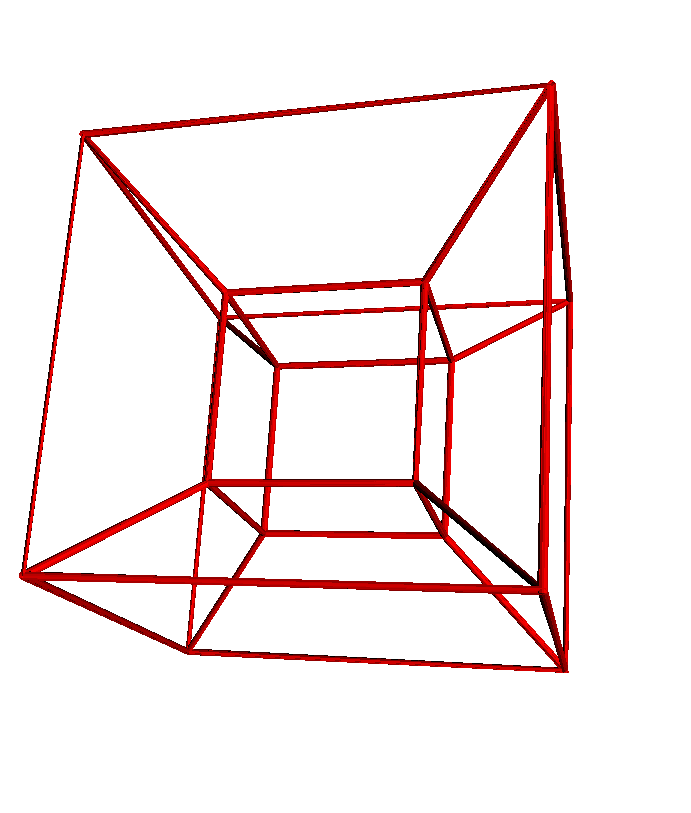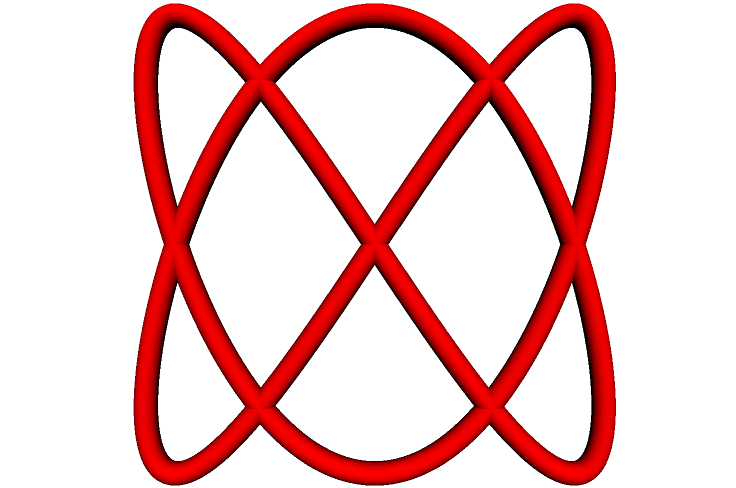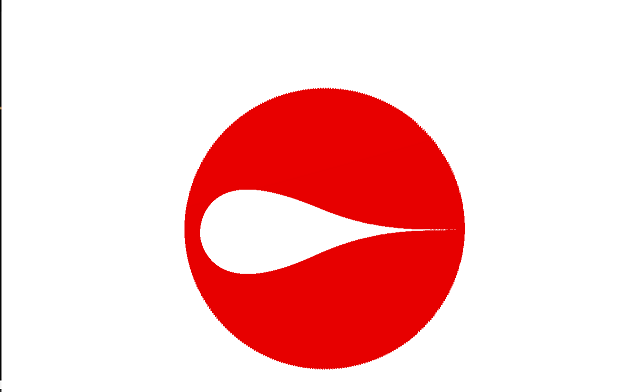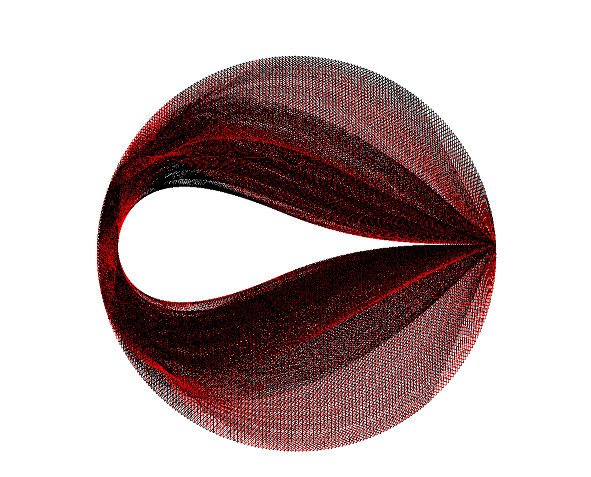# teaching machines

May 20, 2015 by . Filed under cs455, postmortems, spring 2015.

The first thing that I decided to try and make was a hyper cube. I wasn’t to familiar with how the language worked so I ended up just using a bunch of hardcoded moveto commands. I had some trouble figuring out if the push and pop command worked or if I was just using it incorrectly, so  I end up retracing a lot of lines that I would have rather popped back to .to hyperCube =
nsides = 10
moveto 0,0,0
size = 5
for i in 0..4
move size
yaw 90
end
pitch 90
move size
pitch 90
for i in 0..4
move size
yaw 90
end
pitch -90
move size
yaw -90
move size
pitch -90
move size
yaw 90
move size
pitch 90
move size
pitch 90
move -size
outerCubeDiag = 3
moveto 0,0,0

moveto -3,-3,3
moveto 0,0,0
moveto 5,0,0
moveto 8,-3,3
moveto -3,-3,3
moveto 0, 0, 0
moveto 0,0,-5
moveto -3,-3,-8
moveto -3,-3,3
moveto 0,0,0
moveto 0,5,0
moveto -3,8,3
moveto -3,-3,3
moveto 8,-3,3
moveto 8,8,3
moveto 5,5,0
moveto 8,8,3
moveto -3, 8, 3
moveto -3,8,-8
moveto 0,5,-5
moveto -3,8,-8
moveto -3,-3,-8
moveto 8,-3,-8
moveto 5,0,-5
moveto 5,5,-5
moveto 8,8,-8
moveto 8,-3,-8
moveto 8, -3, 3
moveto 8,8,3
moveto 8,8,-8
moveto -3,8,-8

end
hyperCube
tube

Next I decided to mess around with the fibonacci numbers a bit. Some of the other representations of them that came up were cool ,but I thought the pyramid was the most interesting. You can find so many images and shapes in the pyramid as you move around and change the perspective. In a couple instances the pyramid sort of looks like it is coming out of the screen.

to fibPyramid =

nsides = 30

fib = 0
fibStart = 0
fibNext = 1

for t to 25

fib = fibStart + fibNext
fibStart = fibNext
fibNext = fib

moveto fib, 0 , 0
moveto 0, fib, 0
moveto 0, 0, fib
end
end
fibPyramid

After the I  messed around with the fibonacci numbers I decided to turn towards different math equations. I started simple with changing the coordinates by different sin value as it approaches a full circle.to begin =
x = 0.1
y = 0.1
–moveto 0,0,0
nsides = 30
for t to 361
x = sin 2*t
y = sin 3*t
z = sin 7*t
moveto x,y,z
end
end
begin
tube

Once I had the hang of things a bit I decided to find some equations that I thought might look cool. This is utilizing the equation for a tear drop. I tried messing around with the area to create a 3D tear but it didn’t look as good to me.to tearDrop =
x = 0.1
y = 0.1
–moveto 0,0,0
nsides = 30
for t to 361
x = cos t

for m to 7
y = (sin t) * (sin t//2) ^ m
a = (4*(3.14)^0.5) * (0.5*(3+m))//(3+0.5*m)

moveto x,y,0
end

end
end

tearDrop
–fracture = 1

–tube
–dots

surface 361, 7

This is another equation I found that looked cool. My attempt at a Chrysanthemum.

to chrysanthemum =

nsides = 30

for u to 361*2
r = 5 * (1 + sin(11* u // 5)) – 4 * (sin (17 *u // 3))^4 * sin (2 *(cos(3* u))^8 – 28 * u)
x = r * cos u
y = r * sin u

moveto x,y,r
end
end

chrysanthemum

–tube
–dots

surface 361,2

The last one I made isn’t anything specific I know the name of. I was messing around with a few spiral equations for a while and it eventually turned into this.

to spiralStrip =

nsides = 30
r = 5
step = 5
z = 3
a = 0
for a to 10
for t to 361

r = ((a^2) * 1.61)^0.5
x = r * cos t
y = r * sin t
moveto a*x, a*y, t
end
end
end

spiralStrip
–fracture = 1

tube
–surface 361, 10
–dots

–surface 361,2

–revolve 0,0,1,180

Had a lot of fun with this assignment!

p.s. I tried messing with some fractal equations and I don’t know if it is possible, but I think it would be cool if you could switch into a mode that dealt with more continuous functionality. Maybe another side window to script in that manipulates your geometry.